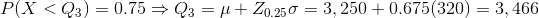# AP Statistics : How to find confidence intervals

## Example Questions

### Example Question #61 : Ap Statistics

Which of the following statements are correct about confidence intervals?

The width of a confidence interval increases as the sample size increases and increases as the confidence level decreases.

The width of a confidence interval increases as the sample size increases and increases as the confidence level increases.

The width of a confidence interval decreases as the sample size increases and increases as the confidence level decreases.

The width of a confidence interval does not change as the sample size increases and increases as the confidence level increases.

The width of a confidence interval decreases as the sample size increases and increases as the confidence level increases.

The width of a confidence interval decreases as the sample size increases and increases as the confidence level increases.

Explanation:

Larger samples give narrower intervals. We are able to estimate a population proportion more precisely with a larger sample size.

As the confidence level increases the width of the confidence interval also increases. A larger confidence level increases the chance that the correct value will be found in the confidence interval. This means that the interval is larger.

### Example Question #62 : Ap Statistics

You are asked to create a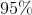confidence interval with a margin of error no larger thanwhile sampling from a normally distributed population with a standard deviation of. What is the minimum required sample size?Explanation:

Keep in mind that the margin of error for a confidence interval based on a normal population is equal to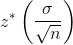, where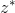is the-score corresponding to the desired confidence level.

From the problem, we can tell that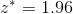and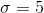. We can then solve foralgebraically: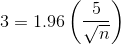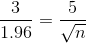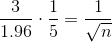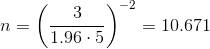The minimum sample size is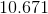rounded up, which is. If you are unsure on problems like these, you can check the margin of error for your answer rounded down and then rounded up (in this case, for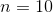and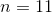.)

### Example Question #63 : Ap Statistics

Jim calculated aconfidence interval for the mean height in inches of boys in his high school. He is not sure how to interpret this interval. Which of the following explains the meaning ofconfidence.of all possible sample means fall within Jim's interval.

In the long run,of all confidence intervals calculated from the same population will contain the true mean height.of boys' heights fall with the interval Jim calculated.

There is achance that Jim's interval contains the true mean height.

In the long run,of all confidence intervals calculated from the same population will contain the true mean height.

Explanation:

95% confidence means that the methods Jim uses to calculate his confidence interval give him correct results 95% of the time. It does not mean that there is a 95% chance that the true mean will be inside the interval. It also does not mean that 95% of all heights or possible sample means fall within the interval.

### Example Question #64 : Ap Statistics

The number of hamburgers served by McGregors per day is normally distributed and has a mean of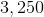hamburgers and a standard deviation of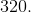Find the range of customers served on the middlepercent of days.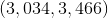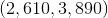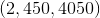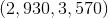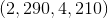Explanation:

First, find the first quartile of the distribution.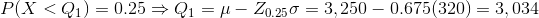Then, find the third quartile of the distribution.